Volume 65 Supplement 11

# Swarm SCARF equatorial electric field inversion chain

## Abstract

The day-time eastward equatorial electric field (EEF) in the ionospheric E-region plays a crucial role in equatorial ionospheric dynamics. It is responsible for driving the equatorial electrojet (EEJ) current system, equatorial vertical ion drifts, and the equatorial ionization anomaly (EIA). Due to its importance, there is much interest in accurately measuring and modeling the EEF for both climatological and near real-time studies. The Swarm satellite mission offers a unique opportunity to estimate the equatorial electric field from measurements of the geomagnetic field. Due to the near-polar orbits of each satellite, the on-board magnetometers record a full profile in latitude of the ionospheric current signatures at satellite altitude. These latitudinal magnetic profiles are then modeled using a first principles approach with empirical climatological inputs specifying the state of the ionosphere. Since the EEF is the primary driver of the low-latitude ionospheric current system, the observed magnetic measurements can then be inverted for the EEF. This paper details the algorithm for recovering the EEF from Swarm geomagnetic field measurements. The equatorial electric field estimates are an official Swarm level-2 product developed within the Swarm SCARF (Satellite Constellation Application Research Facility). They will be made freely available by ESA after the commissioning phase.

## 1. Introduction

Electromagnetic fields in the Earth’s ionosphere are responsible for driving many interesting phenomena. At low and mid latitudes, neutral winds combine with ionospheric electric fields to drive the equatorial electrojet (EEJ) and solar-quiet (Sq) current systems (Sugiura and Poros, 1969; Richmond, 1973) which produce significant magnetic signatures both on the ground and at low Earth orbiting (LEO) satellite altitude. Equatorial electric fields are also responsible for driving the equatorial plasma fountain, which lifts plasma to the upper regions of the ionosphere, where it then diffuses downward and poleward to form enhanced density regions near ±15° magnetic latitude, known as the equatorial ionization anomaly (EIA) (Anderson, 1981). In recent decades, direct measurements of ionospheric electric fields have been restricted to a small number of ground-based radar systems (Hysell et al., 1997; Chau and Woodman, 2004; Chau and Kudeki, 2006) and a few satellite missions (Fejer et al., 2008; de la Beaujardière and the C/NOFS Science Definition Team, 2004). Due to the sparse availability of ionospheric electric field measurements, techniques have been developed over the past decade to indirectly infer electric field values from other sources, in particular at low-latitudes. Anderson et al. (2004) developed a method to infer equatorial vertical ion drift velocities from ground-based magnetometer measurements in Peru using an observatory close to the magnetic equator and another several degrees higher in latitude. Their method relies on training a neural network with horizontal magnetic field inputs ΔH and known electric field outputs which were provided by the Jicamarca radar near Lima, Peru. Without a global set of electric field measurements it is difficult to extend this technique to other longitudes.

Alken and Maus (2010a) developed a technique to estimate the equatorial electric field (EEF) from a latitudinal profile of the EEJ current as the CHAMP satellite crossed the magnetic equator, building upon the earlier work of Lühr et al. (2004). Alken et al. (2013) then extended this work to derive EEF estimates in real-time using ΔH measurements from ground-based magnetometers at any longitude. This method will be used to produce EEF estimates in near real-time each time a Swarm satellite crosses the magnetic equator. While parts of this algorithm have been published before in the previously mentioned papers, the detailed algorithm has never been published in its entirety. The purpose of this paper is to detail the whole algorithm. This so-called “Swarm SCARF equatorial electric field inversion chain” is one of more than a dozen processing chains developed by the Swarm SCARF (Satellite Constellation Application Research Facility) to be operated during the mission (see Olsen et al., 2013), to which IPGP and NOAA will further contribute via the Swarm SCARF dedicated ionospheric and lithospheric chains (see Chulliat et al. (2013) and Thébault et al. (2013)). General information about the Swarm mission can otherwise be found in Friis-Christensen et al. (2006), (2009).

## 2. Satellite Data

The primary input to the equatorial electric field chain will come from the Swarm absolute scalar magnetometer (ASM) instrument on-board all three satellites (Leger et al., 2009). The Swarm ASM is expected to provide 1 Hz scalar magnetic field measurements with an accuracy better than 0.3 nT (Friis-Christensen et al., 2006). While the ASM is also capable of measuring the vector field, only the scalar field data is used for the EEF chain, since the EEJ signature is clearly observable in scalar field measurements and the EEF modeling procedure is considerably simpler with scalar data.

Many of the algorithms in the EEF inversion chain were developed during the CHAMP satellite mission (Reigber et al., 2003). The CHAMP satellite (2000–2010) flew in a near polar orbit (87.3° inclination) with an initial altitude of 454 km which decayed to about 250 km by the end of its mission. CHAMP carried both a scalar Overhauser and a vector fluxgate magnetometer. Many CHAMP-based studies of ionospheric electromagnetic fields and currents led to the development of the algorithms used in the Swarm EEF inversion chain (Lühr et al., 2004; Alken et al., 2008; Alken and Maus, 2010a). The CHAMP database also served as the primary source of input data during the development of the Swarm EEF processor for the Level 2 processing facility.

## 3. Coordinate Systems

The Swarm scalar magnetic measurements will contain contributions from the Earth’s core, lithospheric, ionospheric, and magnetospheric fields. An important step in the processing is to compute scalar magnetic residuals which represent the ionospheric equatorial electrojet, eliminating as many other sources of the geomagnetic field as possible. While this is described in detail in the following section, here we will discuss the various coordinate systems used during this analysis. During the data processing, we fit a model of the Sq (solar-quiet) mid-latitude ionospheric current system to the scalar residuals in order to eliminate its magnetic signature. This model is a standard spherical harmonic expansion in three dimensions, however we replace the geocentric colatitude θ with quasi-dipole colatitude, denoted θq (Richmond, 1995). Quasi-dipole coordinates are a generalization of simple dipole coordinates to a general geomagnetic field. Quasi-dipole latitude is a coordinate which varies along the geomagnetic field B, but changes only slightly with altitude. These coordinates are used because the ionospheric current systems (both Sq and EEJ) are organized with respect to the geomagnetic field, and using a coordinate system which exploits this fact reduces the number of spherical harmonic coefficients needed to model the Sq magnetic field. Throughout the paper, when we refer to the “magnetic equator”, we mean the quasi-dipole equator where the quasi-dipole latitude is 0. The spherical harmonic model used to filter out Sq is also designed to filter out fields originating in the magnetosphere. We represent this part of the model in solar magnetic (SM) coordinates (Russell, 1971). In solar magnetic coordinates, the Z axis is chosen parallel to the Earth’s magnetic dipole and positive toward north. The Y axis is chosen perpendicular to the Earth-Sun line and positive toward dusk. The X axis completes the right-handed basis set and is positive toward the Sun. Since most of the magnetospheric field contribution is driven by solar forcing, using SM coordinates exploits this geometry to reduce the number of external field coefficients needed to sufficiently model these effects. Now that we have defined the relevant coordinate systems for our analysis, we will discuss the processing of the scalar magnetic data in detail.

## 4. Preprocessing

The first step in the data processing involves detection of day-side equatorial crossings. Because the EEJ signal vanishes during the night, or is too weak to perform a meaningful inversion, we restrict our analysis to crossings of the magnetic equator between 06:00 and 18:00 local time. When such a dayside orbit is detected, it is analyzed from −65 to +65 degrees quasi-dipole magnetic latitude. This latitude range is designed to ensure the magnetic signal of both the ionospheric Sq and EEJ current systems are captured. Although we are mainly interested in the EEJ signal, the effect of Sq needs to be carefully separated from the total signal, since Sq effects can be significant at low latitudes where the EEJ is flowing. This is further discussed in the next section.

Next, we remove effects from the core, lithosphere and magnetosphere along the dayside orbit. The total internal field is given by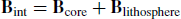(1)

Initially, the POMME-6 magnetic field model (Maus et al., 2006) will specify the core and lithospheric fields, however later in the mission the official Swarm core and lithospheric field models will be used (Rother et al., 2013; Sabaka et al., 2013; Thébault et al., 2013). Defining Fint = ǀBintǀ, we then compute the component of the magnetospheric field and its induced counterpart along the internal field direction: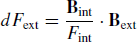(2)

where Bext is the magnetospheric field component of POMME-6, which depends on more than a year of continuous time series inputs of past Dst and F10.7 measurements. We then compute the magnetic scalar residuals along the orbit as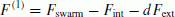(3)

Here, Fs warm are the Level 1b scalar magnetic data measured by the ASM instrument on the Swarm satellite. The residuals F(1) are primarily a combination of the magnetic signatures of the Sq and EEJ current systems, with some possible effects not completely removed by the magnetospheric field model.

## 5. Sq Removal

The next step in the processing is to remove the Sq signal as well as other possible fields that are not described by our magnetospheric model to recover an as clean as possible magnetic signal of the equatorial electrojet current. This is done by fitting a spherical harmonic magnetic field model comprised of fields of both internal and external origin and subtracting it from the residuals. The magnetic potential for current sources below the satellite shell is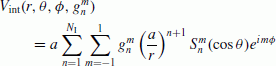(4)

where a is taken to be an Earth radius of 6371.2 km,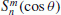are the Schmidt-normalized associated Legendre functions, and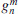are model coefficients to be determined. The magnetic potential for current sources above the satellite shell is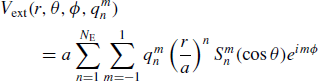(5)

where the external coefficients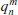are to be determined. NI and NE are the highest spherical harmonic degrees for the internal and external field expansions, and are set to 12 and 2 respectively by the Swarm EEF processor. Only coefficients with order ǀmǀ ≤ 1 are used, since we are fitting a latitudinal profile sampled over a partial orbit, with a limited variation of longitude and altitude, and will use quasi-dipole coordinates (see below) to reduce the number of required coefficients. The internal and external potential coefficients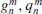are assumed to be time independent for each orbit, since Swarm will not vary significantly in local-time over the course of one orbit. The magnetic fields resulting from the internal and external magnetic potentials are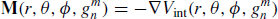(6)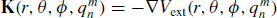(7)

The unknown model coefficients are computed by minimizing the error function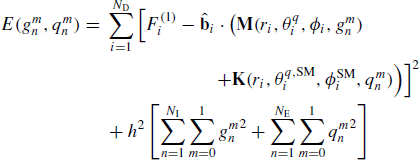(8)

where i is summed over all ND scalar measurements(typically around 2000 measurements sampled at 1 Hz), i is a unit vector in the direction of the internal field Bint at the satellite position i,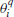is the quasi-dipole colatitude of the measurement location i, andrepresent the point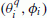transformed into Solar-Magnetic coordinates. h is a damping factor designed to prevent nonphysically large model coefficients in the least-squares solution, and is set by default to 0.001 by the Swarm EEF processor.

After the model coefficients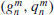are calculated through least-squares inversion, the final magnetic scalar residuals are computed by subtracting the internal and external field models projected onto the main field direction: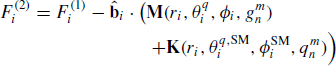(9)

Figure 1 (top) shows a sample magnetic profile of F(1) residuals (solid) recorded by the CHAMP satellite during a single orbit on December 12, 2005 along with the fitted internal and external field model (dashed) as a function of quasi-dipole latitude. We see here the distinctive depression of the main field due to the equatorial electrojet current at the magnetic equator. The sample profile shows the magnetic field behavior of the Sq current system at mid-latitudes during quiet-time, but it is worth noting that the structure of this profile can change substantially during disturbed conditions. The model described above, however, is designed to capture much of this external field variability at mid-latitudes and has proven very robust in accurately estimating the low-latitude EEJ current signature. The bottom panel of Fig. 1 shows the F(2) residuals of the same profile after subtracting the model. The equatorial electrojet peak here is negative since CHAMP is flying well above the current, where the magnetic field due to the EEJ opposes the main field direction.

## 6. Current Inversion

The result of removing the Sq and other residual fields from the scalar magnetometer measurements is assumed to be a clean latitudinal magnetic signature of the equatorial electrojet current for every orbit on the day-side. Our goal is to recover the equatorial electric field driving this current system, and so the next step is to invert the magnetic signature along each track for an estimate of the height-integrated EEJ current. To do this, we define a simple sheet current model of N C linear currents flowing longitudinally eastward along lines of constant quasi-dipole latitude and an altitude of 110 km. This simple model is designed to represent the geomagnetically eastward flowing EEJ current which peaks in the E-region at about 110 km altitude (Heelis, 2004). The N C currents are spaced equally within a 30 degree band in geocentric colatitude, where the colati-tude directioncorresponds to magnetic south at the location of the magnetic equator crossing (see Fig. 2). This band is suited to capture the low-latitude current aligned with the magnetic equator. A more sophisticated model would allow for currents flowing at different altitudes, allowing us to recover the full latitudinal and radial dependence of the EEJ current system. However, a single latitudinal magnetic profile as measured by the satellite crossing the magnetic equator above the EEJ system is not sufficient to uniquely constrain the altitude dependence of such a model. We are therefore only able to estimate the height-integrated eastward current flowing at a specific latitude. While the true EEJ system is best represented as a curved current arc at a constant altitude, for ease of processing we divide each of the N C currents into 360 segments, where each segment is a straight-line current spanning 1 degree of geographic longitude, and the endpoints of each segment are located at 110 km altitude. Figure 2 shows a sketch of the geometry of the current model and other relevant parameters discussed below.

The unit current vector for longitude segmentk(1 … 360) of current j(1 … N C ) is then given by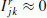(10)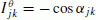(11)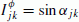(12)

where α jk is the angle between geographic north and the linear current segment k of current j. The unit current vector I jk will later be multiplied by a current strength S j , with units of amperes, which is to be determined using the satellite magnetic residuals. To determine the unknown current strengths from the scalar magnetic residuals, we compute the magnetic field at satellite observation point i, due to line segment k of arc current j using the Biot-Savart law: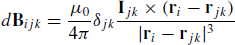(13)

where δ jk is the distance in meters of segment k of current j, r i is the position vector of satellite observation i, and r jk is the position vector pointing to the midpoint of line segment k of arc current j. To determine the total unit magnetic field contribution from arc current j at satellite observation point i, we can sum over longitudinal segments k:(14)

Not all of the 360 longitudinal segments are used in this sum, since the EEJ current flowing far away from the satellite has less influence on the measured magnetic field. We therefore include longitudinal segments only within ±30 degrees geographic longitude of the satellite’s crossing of the magnetic equator in the above sum. Since we are using only the scalar magnetometer measurements on-board Swarm, we project B ij onto the internal field direction using the POMME model:(15)

Then, the unknown arc current strengths S j representing the equatorial electrojet current model can be calculated by least-squares minimization of the error function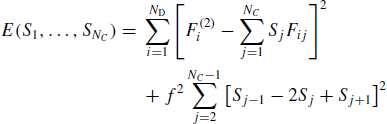(16)

where the first sum over j represents the fit to the total scalar field from all N C arc currents in our EEJ model, and the second term is designed to damp the solution so that neighboring arc currents do not vary wildly in magnitude. This helps to ensure a smooth latitudinal current profile solution. By default, the Swarm EEF processor sets the damping factor f = 5.

Once the current strengths S j have been determined, the total height-integrated eastward current density flowing at 110 km altitude is calculated at each latitude by dividing the corresponding current strength by the latitudinal distance d in meters between arc currents: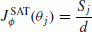(17)

The Swarm EEF processor defines a latitudinal spacing between arc currents of 0.5 degrees, leading to a distance d = 56.6 km. The ϕ direction of Eq. (17) is the direction of magnetic east at the location of the satellite crossing the magnetic equator (see Fig. 2). The height-integrated current density has units of A/m. Figure 3 shows an example height-integrated current profile from the inversion of the magnetic measurements shown in Fig. 1. We see here the peak current flow at the magnetic equator as well as some interesting structure at higher latitudes. The peak at 0 degrees quasi-dipole latitude is primarily due to the equatorial electric field strength, while the higher latitude structure is primarily due to the neutral wind field (Fambitakoye et al., 1976, p. 114). In the next section, we will discuss the procedure for recovering the EEF by modeling these height-integrated current profiles.

## 7. Electrodynamic Modeling

The electrostatic fields and currents of the ionosphere are governed by the equations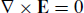(18)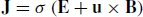(19)

where E is the electric field, J is the current density, σ is the anisotropic conductivity tensor (Forbes, 1981, eq. 10), u is the neutral wind velocity field, and B is the ambient geomagnetic field. Equation (18) is Faraday’s law in a steady-state magnetic field and Eq. (19) is Ohm’s law describing the current density driven by the neutral winds and electric field. We solve these equations in spherical geocentric coordinates, however the coordinates are rotated so that the azimuthal direction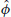is tangent to the magnetic equator at the location of the satellite crossing (see Fig. 2). This is a first-order correction in order to allow the modeling of the currents flowing along lines of constant quasi-dipole latitude, as we calculated during the satellite data inversion step (Eq. (17)). To perform a strict comparison between our modeled current and Eq. (17) would require solving the electrostatic equations in quasi-dipole coordinates, but this first order correction enables us to use the simplicity of spherical coordinates and captures most of the difference between magnetic and geographic east. Next, we assume that the longitudinal gradients of all terms vanish (/ φ = 0). This assumption is known to be incorrect on large scales, particularly at the boundaries of the 4-cell non-migrating ionospheric structure, where gradients in E × B drift velocities have been reported of up to 3 m/s/deg (Araujo-Pradere et al., 2011). To fully account for these effects, we would need to solve the electrostatic equations in three dimensions. However, previous calculations of electric fields ignoring longitudinal gradients have demonstrated remarkable agreement with radar measurements at Jicamarca (Alken and Maus, 2010a). This assumption, with the condition J = 0, allows the J r and J θ components to be derived from a single current stream function ψ (Sugiura and Poros, 1969):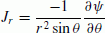(20)(21)

Equation (18) becomes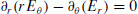(22)(23)

where R is a constant of integration and can be taken as a reference radius, and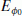is the eastward electric field at the equator at the radius R. Equation (23) shows that for a given value of the equatorial eastward electric field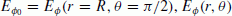is determined everywhere in the (r, θ) plane. The unknowns to be determined are therefore E r , E θ , and ψ. We use empirical models to supply the conductivity σ, wind field u and geomagnetic field B. The conductivity requires knowledge of the global densities and temperatures of the electrons, ions and neutrals. For these we use the IRI-2012 (Bilitza et al., 2011) and NRLMSISE-00 (Picone et al., 2002) models. The equations for the direct, Pedersen and Hall conductivities are given in Kelley (1989), (appendix B). The neutral wind field u is supplied by the Horizontal Wind Model (HWM07) (Drob et al., 2008; Emmert et al., 2008). HWM07 does not provide vertical wind velocities, and so they are ignored during this modeling. The geomagnetic field B is specified by the POMME-6 main field model (Maus et al., 2006).

Eliminating E r and E θ from Eqs. (19)–(22) yields a second order partial differential equation (PDE) for the current stream function ψ: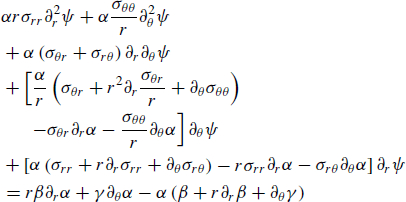(24)

where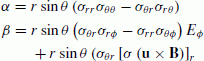(25)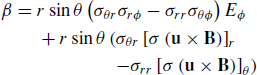(26)(27)

and the conductivity tensor σ is represented in a basis of spherical coordinates. The components of the conductivity tensor may be related to the direct, Pedersen, and Hall conductivities using Richmond (1995), (eq. 2.1). The direct, Pedersen, and Hall conductivities are given in Kelley (1989), (appendix B), and are reproduced below: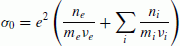(28)(29)(30)

Here, the i sums over all ion species in the ionosphere. e is the electron charge, n e is the electron density, n i is the ion density of species i, m e and m i are the electron and ion masses, v e and v i are the electron and ion collision frequencies, and Ω e and Ω i are the electron and ion gyro-frequencies around the magnetic field lines. Expressions for the collision frequencies v e and v i are given in Kelley (1989), (appendix B). The ionospheric densities and temperatures are taken from IRI-2012. The neutral density needed to compute the collision frequencies is taken from the NRLMSISE-00 model. Previous efforts to model the equatorial electrojet have found it necessary to increase the electron collision frequency v e by an empirical factor of 4 during typical day-time eastward electric field conditions, to account for unmodeled nonlinear instabilities in the electrojet stream (Gagnepain et al., 1977; Ronchi et al., 1990, 1991; Fang et al, 2008; Alken and Maus, 2010a, b). We adopt this same convention for the Swarm EEF chain when calculating the conductivities.

The PDE in Eq. (24) is solved on a 2D grid in the (r, θ) plane, holding ϕ fixed at the longitude of the satellite crossing of the magnetic equator. The grid ranges from 65 to 500 km altitude in steps of 2.175 km, and −25 to 25 degrees latitude in steps of 0.25 degrees. The boundary conditions imposed on the PDE are that the current vanishes at the lower and upper boundaries (ψ = 0 at r = rmin and rmax), and there is no radial current flow at the northern and southern boundaries (at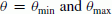and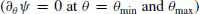). We solve the PDE using finite differencing on the 2D grid with a 9-cell stencil.

## 8. EEF Inversion

In Eqs. (26)–(27), the terms θ and γ depend on the eastward equatorial electric field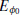, which we are seeking through this modeling process. Our approach will be to use an initial guess for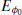and then use the satellite-derived current (Eq. (17)) to refine the guess until the misfit between the modeled and observed current is minimized. This problem can be made linear by noting from Eq. (19) that the current density is linear in E when the wind field u = 0. Defining the height-integrated eastward current calculated from our model as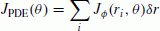(31)

where δr is the radial grid spacing, the final eastward electric field is computed from a least squares inversion of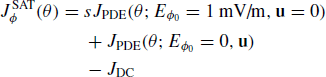(32)

In the right hand side of Eq. (32) we have separated the modeled current solution into two pieces, the first in which we set the wind field u = 0 and use an initial guess of 1 mV/m for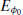, and the second in which we allow the wind field and turn off the eastward electric field. The first term is then linear in, allowing us to solve for a scaling factor s to minimize the misfit with the satellite-derived current. Therefore, the PDE in Eq. (24) is solved twice, once with the eastward electric field turned off, and once with the winds turned off. The final eastward equatorial electric field (EEF) is then s∙ (1 mV/m). Jdc is a constant DC offset to allow for a difference in zero levels between the modeled and observed current. The parameters s and Jdc are determined by least-squares inversion of Eq. (32), with the additional constraint that the left and right hand sides of that equation must agree at the magnetic equator (θ = π/2). This constraint has been found to yield more accurate electric fields (Alken and Maus, 2010a), since the EEF is primarily responsible for current structure near the magnetic equator, while the winds are primarily responsible for current structure at higher latitudes (Fambitakoye et al, 1976).

Figure 4 shows the modeled height-integrated current density (dashed) derived from the CHAMP current profile (solid) also shown in Fig. 3. We can see that the two profiles agree at the magnetic equator, since this condition was imposed on the least-squares inversion. The main peak is modeled fairly well, while the sidelobes exhibit more disagreement. This is fairly typical in this modeling approach since the sidelobes are primarily determined by the wind field at both E and F region altitudes, and the climatologi-cal Horizontal Wind Model cannot capture the full day-today variability of the winds. Luckily, the main peak size and shape is primarily determined by the equatorial zonal electric field (with some possible contribution from E region vertical winds which are not modeled). Therefore, accurately modeling this peak will produce reliable estimates of the EEF. In the next section we will discuss validation of the EEF estimates against independent observations.

## 9. Validation of EEF Estimates

To obtain an estimate of the error in the EEF calculated from a given orbit, we use the relative error between the satellite-derived and modeled current profiles: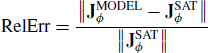(33)

where the components of the vector quantities above correspond to different colatitudes θ, and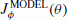is the right hand side of Eq. (32). This relative error value is provided as an output in the Level 2 Swarm EEF data files, and is an indication of the quality of the corresponding EEF estimate. Small (< 1.0) values of RelErr indicate good agreement between the observed and modeled current and therefore more accurate estimates of the EEF. Large values (>1.0) indicate that the modeling was unable to reproduce important features of the satellite-derived current. This could be due to periods of high disturbance which cannot be adequately captured by climatological modeling, or possibly due to local-times where the current signal is very weak. We applied the EEF chain algorithm to all CHAMP satellite data from May 2001 through June 2007 and found 81.7% of the dataset had a relative error less than 1, and 18.3% of the profiles had a value greater than 1.

A primary independent source of equatorial electric field measurements comes from the JULIA radar located at the Jicamarca Radio Observatory near Lima, Peru. JULIA (Jicamarca Unattended Long Term Investigations of the Atmosphere) is a coherent scatter radar which measures the velocity of upward drifting plasma near 150 km altitude (Chau and Woodman, 2004; Chau and Kudeki, 2006). This vertical drift is caused by the eastward equatorial electric field, and so the vertical drift velocity directly yields the eastward electric field through the relation E = −v × B. We compared EEF estimates from direct CHAMP overflights of Jicamarca with JULIA measurements. A direct overflight was defined as CHAMP passing within ±10 degrees longitude of Jicamarca and within 5 minutes of a corresponding JULIA measurement. These data pairs are plotted in Fig. 5. A total of 162 data pairs were found for the period May 2001-June 2007. In red are plotted the data corresponding to a relative error less than 1 in the EEF modeling. The blue points correspond to a relative error greater than 1. While there are only 13 points with a large relative error, we see that these satellite EEF estimates still agree very well with JULIA measurements. The correlation between the satellite-derived EEF and JULIA measurements is 0.80, with a best fit line of Echamp = 1.04 × Ejulia + 0.01 mV/m. The y = x line is shown in green. These results demonstrate that the use of climatological models in inverting the satellite magnetic measurements does not lead to a significant suppression of the day-to-day variability. Periodically throughout the Swarm mission, we plan to validate the Level 2 EEF product against JULIA radar measurements for each satellite to ensure the algorithm continues to operate accurately under a wide range of solar conditions.

## 10. Conclusion

In this paper we have presented in detail the algorithm for the Swarm Level 2 Equatorial Electric Field inversion chain. The main inputs to the algorithm are the scalar magnetic field measurements from the absolute scalar magnetometer (ASM) instrument on-board each Swarm satellite. The chain then subtracts internal and magnetospheric field models, filters out the mid-latitude Sq current system, inverts the resulting magnetic profile for the E-region height-integrated current density, and then models this current density with a combination of first-principles and empirical modeling to recover the driving zonal electric field at the time of the satellite crossing of the magnetic equator. This algorithm has been thoroughly tested against the CHAMP database, and the resulting EEF estimates have been validated against independent measurements from the JULIA radar, with a correlation of 0.80 between the two, a best fit line with a slope of nearly 1, and a bias close to 0. Validation against JULIA measurements will be carried out periodically throughout the Swarm mission to ensure the algorithm continues to produce reliable EEF estimates.

## References

1. Alken, P. and S. Maus, Electric fields in the equatorial ionosphere derived from CHAMP satellite magnetic field measurements, J. Atmos. Sol. Terr. Phys., 72, 319–326, doi:10.1016/j.jastp.2009.02.006, 2010a.

2. Alken, P. and S. Maus, Relationship between the ionospheric eastward electric field and the equatorial electrojet, Geophys. Res. Lett., 37, L04104, doi:10.1029/2009GL041989, 2010b.

3. Alken, P., S. Maus, J. Emmert, and D. P. Drob, Improved horizontal wind model HWM07 enables estimation of equatorial ionospheric electric fields from satellite magnetic measurements, Geophys. Res. Lett., 35, L11105, doi:10.1029/2008GL033580, 2008.

4. Alken, P., A. Chulliat, and S. Maus, Longitudinal and seasonal structure of the ionospheric equatorial electric field, J. Geophys. Res., 118, doi:10.1029/2012JA018314, 2013.

5. Anderson, D. N., Modeling the ambient, low latitude F-region ionosphere—a review, J. Atmos. Terr. Phys., 43(8), 753–762, 1981.

6. Anderson, D., A. Anghel, J. Chau, and O. Veliz, Daytime vertical E × B drift velocities inferred from gound-based magnetometer observations at low latitudes, Space Weather, 2, S11001, doi:10.1029/2004SW000095, 2004.

7. Araujo-Pradere, E. A., D. N. Anderson, and M. Fedrizzi, Communications/Navigation Outage Forecasting System observational support for the equatorial E × B drift velocities associated with the four-cell tidal structures, Radio Sci., 46, RS0D09, doi:10.1029/2010RS004557, 2011.

8. Bilitza, D., L.-A. McKinnell, B. Reinisch, and T. Fuller-Rowell, The International Reference Ionosphere (IRI) today and in the future, J. Geod., 85, 909–920, doi:10.1007/s00190-010-0427-x, 2011.

9. Chau, J. L. and E. Kudeki, Statistics of 150-km echoes over Jicamarca based on low-power VHF observations, Ann. Geophys., 24, 1305–1310, 2006.

10. Chau, J. L. and R. F. Woodman, Daytime vertical and zonal velocities from 150-km echoes: Their relevance to F-region dynamics, Geophys. Res. Lett., 31, L17801, doi:10.1029/2004GL020800, 2004.

11. Chulliat, A., P. Vigneron, E. Thébault, O. Sirol, and G. Hulot, Swarm SCARF Dedicated Ionospheric Field Inversion chain, Earth Planets Space, 65, this issue, 1271–1283, 2013.

12. de la Beaujardière, O. and the C/NOFS Science Definition Team, C/NOFS: A mission to forecast scintillations, J. Atmos. Sol. Terr. Phys., 66(17), 1573–1591, doi:10.1016/j.jastp.2004.07.030, 2004.

13. Drob, D. P. et al., An empirical model of the Earth’s horizontal wind fields: HWM07, J. Geophys. Res., 113, A12304, doi:10.1029/2008JA013668, 2008.

14. Emmert, J. T., D. P. Drob, G. G. Shepherd, G. Hernandez, M. J. Jarvis, J. W. Meriwether, R. J. Niciejewski, D. P. Sipler, and C. A. Tepley, DWM07 global empirical model of upper thermospheric |storm-induced disturbance winds, J. Geophys. Res., 113, A11319, doi:10.1029/2008JA013541, 2008.

15. Fambitakoye, O., P. N. Mayaud, and A. D. Richmond, The equatorial electrojet and regular daily variation S R :-III. Comparison of observations with a physical model, J. Atmos. Terr. Phys., 38, 113–121, 1976.

16. Fang, T. W., A. D. Richmond, J. Y. Liu, A. Maute, C. H. Lin, C. H. Chen, and B. Harper, Model simulation of the equatorial electrojet in the Peruvian and Philippine sectors, J. Atmos. Sol. Terr. Phys., 70, 2203–2211, 2008.

17. Fejer, B. G., J. W. Jensen, and S.-Y. Su, Quiet-time equatorial F region vertical plasma drift model derived from ROCSAT-1 observations, J. Geophys. Res., 113, A05304, doi:10.1029/2007JA012801, 2008.

18. Forbes, J. M., The equatorial electrojet, Rev. Geophys. Space Phys., 19(3), 469–504, 1981.

19. Friis-Christensen, E., H. Lühr, and G. Hulot, Swarm: A constellation to study the Earth’s magnetic field, Earth Planets Space, 58, 351–358, 2006.

20. Friis-Christensen, E., H. Lühr, G. Hulot, R. Haagmans, and M. Purucker, Geomagnetic research from space, Eos, 90, 213–214, 2009.

21. Gagnepain, J., M. Crochet, and A. D. Richmond, Comparison of equatorial electrojet models, J. Atmos. Terr. Phys., 39, 1119–1124, 1977.

22. Heelis, R. A., Electrodynamics in the low and middle latitude ionosphere: A tutorial, J. Atmos. Sol. Terr. Phys., 66, 825–38, 2004.

23. Hysell, D. L., M. F. Larsen, and R. F. Woodman, JULIA radar studies of electric fields in the equatorial electrojet, Geophys. Res. Lett., 24(13), 1687–90, 1997.

24. Kelley, M. C, The Earth’s Ionosphere: Plasma Physics and Electrodynamics, Academic Press Inc, San Diego, 1989.

25. Leger, J.-M., F. Bertrand, T. Jager, M. Le Prado, I. Fratter, and J.-C. Lalaurie, Swarm absolute scalar and vector magnetometer based on helium 4 optical pumping, Procedia Chemistry, 1(1), 634–637, 2009, ISSN 1876–6196, doi:10.1016/j.proche.2009.07.158, Proceedings of the Eurosensors XXIII conference.

26. Lühr, H., S. Maus, and M. Rother, Noon-time equatorial electrojet: Its spatial features as determined by the CHAMP satellite, J. Geophys. Res., 109, A01306, doi:10.1029/2002JA009656, 2004.

27. Maus, S., M. Rother, C. Stolle, W Mai, S. Choi, H. Lühr, D. Cooke, and C. Roth, Third generation of the Potsdam Magnetic Model of the Earth (POMME), Geochem. Geophys. Geosyst., 7, doi:10.1029/2006GC001269, 2006.

28. Olsen, N., E. Friis-Christensen, R. Floberghagen, P. Alken, C. D Beggan, A. Chulliat, E. Doornbos, J. T. da Encarnação, B. Hamilton, G. Hulot, J. van den IJssel, A. Kuvshinov, V. Lesur, H. Lühr, S. Macmillan, S. Maus, M. Noja, P. E. H. Olsen, J. Park, G. Plank, C. Püthe, J. Rauberg, P. Ritter, M. Rother, T. J. Sabaka, R. Schachtschneider, O. Sirol, C. Stolle, E. Thébault, A. W. P. Thomson, L. Tøffner-Clausen, J. Veí?mský, P. Vigneron, and P. N. Visser, The Swarm Satellite Constellation Application and Research Facility (SCARF) and Swarm data products, Earth Planets Space, 65, this issue, 1189–1200, 2013.

29. Picone, J. M., A. E. Hedin, D. P. Drob, and A. C. Aikin, NRLMSISE-00 empirical model of the atmosphere: Statistical comparisons and scientific issues, J. Geophys. Res., 107, doi:10.1029/2002JA009430, 2002.

30. Reigber, C, H. Lühr, and P. Schwintzer, First CHAMP Mission Results for Gravity, Magnetic and Atmospheric Studies, Springer, 2003.

31. Richmond, A. D., Equatorial electrojet—I. Development of a model including winds and electric field, J. Atmos. Terr. Phys., 35, 1083–1103, 1973.

32. Richmond, A. D., Ionospheric electrodynamics using magnetic apex coordinates, J. Geomag. Geoelectr, 47, 191–212, 1995.

33. Ronchi, C., R. N. Sudan, and P. L. Similon, Effect of short-scale turbulence on kilometer wavelength irregularities in the equatorial electrojet, J. Geophys. Res., 95(A1), 189–200, 1990.

34. Ronchi, C, R. N. Sudan, and D. T. Farley, Numerical simulations of large-scale plasma turbulence in the daytime equatorial electrojet, J. Geophys. Res., 96(A12), 21,263–21,279, 1991.

35. Rother, M., V. Lesur, and R. Schachtschneider, An algorithm for deriving core magnetic field models from the Swarm data set, Earth Planets Space, 65, this issue, 1223–1231, 2013.

36. Russell, C. T., Geophysical coordinate transformations, Cosmic Electrodynamics, 2, 1971.

37. Sabaka, T. J., L. Tøffner-Clausen, and N. Olsen, Use of the Comprehensive Inversion method for Swarm satellite data analysis, Earth Planets Space, 65, this issue, 1201–1222, 2013.

38. Sugiura, M. and D. J. Poros, An improved model equatorial electrojet with a meridional current system, J. Geophys. Res., 74, 4025–4034, 1969.

39. Thébault, E., P. Vigneron, S. Maus, A. Chulliat, O. Sirol, and G. Hulot, Swarm SCARF Dedicated Lithospheric Field Inversion chain, Earth Planets Space, 65, this issue, 1257–1270, 2013.

## Acknowledgments

The operational support of the CHAMP mission by the German Aerospace Center (DLR) is gratefully acknowledged. The Jicamarca Radio Observatory is a facility of the Instituto Geofisico del Peru operated with support from the NSF through Cornell University. The authors gratefully acknowledge support from the Centre National d’Études Spatiales (CNES) within the context of the “Travaux préparatoires et exploitation de la mission SWARM” project, and from the European Space Agency (ESA) through ESTEC contract number 4000102140/10/NL/JA “Development of the Swarm Level 2 Algorithms and Associated Level 2 Processing Facility.” We also thank two anonymous reviewers for their comments on an earlier version of the manuscript. This is IPGP contribution number 3446.

## Author information

Authors

### Corresponding author

Correspondence to Patrick Alken.

## Rights and permissions

The images or other third party material in this article are included in the article’s Creative Commons licence, unless indicated otherwise in a credit line to the material. If material is not included in the article’s Creative Commons licence and your intended use is not permitted by statutory regulation or exceeds the permitted use, you will need to obtain permission directly from the copyright holder.

To view a copy of this licence, visit https://creativecommons.org/licenses/by/4.0/.

Reprints and Permissions

Alken, P., Maus, S., Vigneron, P. et al. Swarm SCARF equatorial electric field inversion chain. Earth Planet Sp 65, 11 (2013). https://doi.org/10.5047/eps.2013.09.008

• Revised:

• Accepted:

• Published:

### Key words

• Equatorial ionosphere
• electric fields
• space magnetometry
• Swarm